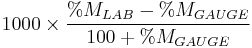# 106.3.2.35 TM-35, Moisture Offset Factor for a Nuclear GaugeCrew takes a soil sample on an interstate to determine soil conditions and how much rock is beneath the surface.

This test determines the moisture offset factor to be applied to the calibration curve values for moisture content determinations by nuclear gauges in soil, soil-aggregate and crushed stone bases.

## 106.3.2.35.1 Equipment

(a) Nuclear moisture-density gauges meeting the apparatus and precision requirements of AASHTO T310.

(b) Equipment specified by AASHTO T205, paragraphs 2.2, 2.3 and 2.4.

## 106.3.2.35.2 Procedure

A correction factor for use with the moisture calibration curve shall be determined as follows:

(1) No fewer than four testsa are required to establish a moisture offset factor. These tests should be obtained from material typical of that to be tested and should be randomly selected over the largest practical area of material positively identifiable as the material to be tested. For soils, the tests may be performed in cuts behind the earth movers.

a The required number of tests is based upon statistical considerations which include a selected confidence level of 99%, a selected confidence interval of 0.5 pcf and a standard deviation of 0.35 pcf for nuclear moisture test values as determined by Research Study 74-2.

(2) At each random site, perform a nuclear wet density and moisture test in accordance with AASHTO T310 Direct Transmission and obtain a moisture sample for heat drying. Obtain the moisture sample and heat dry as follows:

a. Select the sample from that material located between source and detectors during the nuclear determinations.
b. The moisture sample should weigh from 1000 g (2.2 lb) to 1500 g (3.3 lb). The higher weight sample should be obtained when particles larger than 1/4 in. are present. The sample should be obtained to a depth of 5 in. (127 mm) except that the depth should be reduced so as to not exceed the thickness of any layer under test. (Note: The nuclear meter has not been evaluated in crushed stone bases where the lift was less than 4 in. thick.)
c. Determine the moisture content of the total sample by heat drying at 110°C ± 5°C (230°F ± 9°F).

## 106.3.2.35.3 Calculations

(a) Determine the moisture offset factor from the average results of the 4 tests, as follows from Troxler Manual:

K =$1000 \times \frac {%M_{LAB} - % M_{GAUGE}}{100 + %M_{GAUGE}}$
Where:
K = Moisture Offset Factor
% MLAB = Percent moisture of heat dried sample
% MGAUGE = Percent moisture of nuclear gauge

(b) Enter moisture offset factor (K) into gauge as outlined in the Operator’s Manual.

(c) The offset factor can be ignored if 0.5 pcf or less.